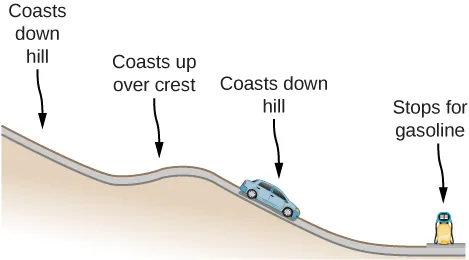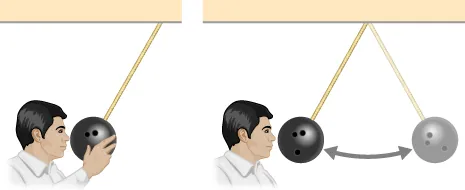University Physics Volume 1

# Conceptual Questions

University Physics Volume 1Conceptual Questions

### 8.1Potential Energy of a System

1.

The kinetic energy of a system must always be positive or zero. Explain whether this is true for the potential energy of a system.

2.

The force exerted by a diving board is conservative, provided the internal friction is negligible. Assuming friction is negligible, describe changes in the potential energy of a diving board as a swimmer drives from it, starting just before the swimmer steps on the board until just after their feet leave it.

3.

Describe the gravitational potential energy transfers and transformations for a javelin, starting from the point at which an athlete picks up the javelin and ending when the javelin is stuck into the ground after being thrown.

4.

A couple of soccer balls of equal mass are kicked off the ground at the same speed but at different angles. Soccer ball A is kicked off at an angle slightly above the horizontal, whereas ball B is kicked slightly below the vertical. How do each of the following compare for ball A and ball B? (a) The initial kinetic energy and (b) the change in gravitational potential energy from the ground to the highest point? If the energy in part (a) differs from part (b), explain why there is a difference between the two energies.

5.

What is the dominant factor that affects the speed of an object that started from rest down a frictionless incline if the only work done on the object is from gravitational forces?

6.

Two people observe a leaf falling from a tree. One person is standing on a ladder and the other is on the ground. If each person were to compare the energy of the leaf observed, would each person find the following to be the same or different for the leaf, from the point where it falls off the tree to when it hits the ground: (a) the kinetic energy of the leaf; (b) the change in gravitational potential energy; (c) the final gravitational potential energy?

### 8.2Conservative and Non-Conservative Forces

7.

What is the physical meaning of a non-conservative force?

8.

A bottle rocket is shot straight up in the air with a speed $30m/s30m/s$. If the air resistance is ignored, the bottle would go up to a height of approximately $46m46m$. However, the rocket goes up to only $35m35m$ before returning to the ground. What happened? Explain, giving only a qualitative response.

9.

An external force acts on a particle during a trip from one point to another and back to that same point. This particle is only effected by conservative forces. Does this particle’s kinetic energy and potential energy change as a result of this trip?

### 8.3Conservation of Energy

10.

When a body slides down an inclined plane, does the work of friction depend on the body’s initial speed? Answer the same question for a body sliding down a curved surface.

11.

Consider the following scenario. A car for which friction is not negligible accelerates from rest down a hill, running out of gasoline after a short distance (see below). The driver lets the car coast farther down the hill, then up and over a small crest. He then coasts down that hill into a gas station, where he brakes to a stop and fills the tank with gasoline. Identify the forms of energy the car has, and how they are changed and transferred in this series of events.12.

A dropped ball bounces to one-half its original height. Discuss the energy transformations that take place.

13.

$E=K+UE=K+U$ constant is a special case of the work-energy theorem.” Discuss this statement.

14.

In a common physics demonstration, a bowling ball is suspended from the ceiling by a rope.

The professor pulls the ball away from its equilibrium position and holds it adjacent to his nose, as shown below. He releases the ball so that it swings directly away from him. Does he get struck by the ball on its return swing? What is he trying to show in this demonstration?15.

A child jumps up and down on a bed, reaching a higher height after each bounce. Explain how the child can increase his maximum gravitational potential energy with each bounce.

16.

Can a non-conservative force increase the mechanical energy of the system?

17.

Neglecting air resistance, how much would I have to raise the vertical height if I wanted to double the impact speed of a falling object?

18.

A box is dropped onto a spring at its equilibrium position. The spring compresses with the box attached and comes to rest. Since the spring is in the vertical position, does the change in the gravitational potential energy of the box while the spring is compressing need to be considered in this problem?

Order a print copy

As an Amazon Associate we earn from qualifying purchases.